The OpenFOAM Foundation
C2H6 Class Reference

ethane More...

Inheritance diagram for C2H6: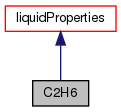[legend]
Collaboration diagram for C2H6:[legend]

## Public Member Functions

TypeName ("C2H6")
Runtime type information. More...

C2H6 ()
Construct null. More...

C2H6 (const liquidProperties &l, const NSRDSfunc5 &density, const NSRDSfunc1 &vapourPressure, const NSRDSfunc6 &heatOfVapourisation, const NSRDSfunc14 &heatCapacity, const NSRDSfunc0 &enthalpy, const NSRDSfunc7 &idealGasHeatCapacity, const NSRDSfunc4 &secondVirialCoeff, const NSRDSfunc1 &dynamicViscosity, const NSRDSfunc2 &vapourDynamicViscosity, const NSRDSfunc0 &thermalConductivity, const NSRDSfunc2 &vapourThermalConductivity, const NSRDSfunc6 &surfaceTension, const APIdiffCoefFunc &vapourDiffussivity)
Construct from components. More...

C2H6 (const dictionary &dict)
Construct from dictionary. More...

virtual autoPtr< liquidPropertiesclone () const
Construct and return clone. More...

scalar rho (scalar p, scalar T) const
Liquid density [kg/m^3]. More...

scalar pv (scalar p, scalar T) const
Vapour pressure [Pa]. More...

scalar hl (scalar p, scalar T) const
Heat of vapourisation [J/kg]. More...

scalar Cp (scalar p, scalar T) const
Liquid heat capacity [J/(kg K)]. More...

scalar h (scalar p, scalar T) const
Liquid Enthalpy [J/(kg)]. More...

scalar Cpg (scalar p, scalar T) const
Ideal gas heat capacity [J/(kg K)]. More...

scalar B (scalar p, scalar T) const
Second Virial Coefficient [m^3/kg]. More...

scalar mu (scalar p, scalar T) const
Liquid viscosity [Pa s]. More...

scalar mug (scalar p, scalar T) const
Vapour viscosity [Pa s]. More...

scalar kappa (scalar p, scalar T) const
Liquid thermal conductivity [W/(m K)]. More...

scalar kappag (scalar p, scalar T) const
Vapour thermal conductivity [W/(m K)]. More...

scalar sigma (scalar p, scalar T) const
Surface tension [N/m]. More...

scalar D (scalar p, scalar T) const
Vapour diffussivity [m2/s]. More...

scalar D (scalar p, scalar T, scalar Wb) const
Vapour diffussivity [m2/s] with specified binary pair. More...

void writeData (Ostream &os) const
Write the function coefficients. More...Public Member Functions inherited from liquidProperties
TypeName ("liquid")

declareRunTimeSelectionTable (autoPtr, liquidProperties,,(),())

declareRunTimeSelectionTable (autoPtr, liquidProperties, dictionary,(const dictionary &dict),(dict))

liquidProperties (scalar W, scalar Tc, scalar Pc, scalar Vc, scalar Zc, scalar Tt, scalar Pt, scalar Tb, scalar dipm, scalar omega, scalar delta)
Construct from components. More...

liquidProperties (const dictionary &dict)
Construct from dictionary. More...

virtual ~liquidProperties ()
Destructor. More...

scalar Y () const
No of moles of this species in mixture. More...

scalar Tc () const
Critical temperature [K]. More...

scalar Pc () const
Critical pressure [Pa]. More...

scalar Vc () const
Critical volume [m^3/kmol]. More...

scalar Zc () const
Critical compressibilty factor. More...

scalar Tt () const
Triple point temperature [K]. More...

scalar Pt () const
Triple point pressure [Pa]. More...

scalar Tb () const
Normal boiling temperature [K]. More...

scalar dipm () const
Dipole moment []. More...

scalar omega () const
Pitzer's ascentric factor []. More...

scalar delta () const
Solubility parameter [(J/m^3)^(1/2)]. More...

scalar limit (const scalar T) const
Limit the temperature to be in the range Tlow_ to Thigh_. More...

scalar psi (scalar p, scalar T) const
Liquid compressibility rho/p [s^2/m^2]. More...

scalar CpMCv (scalar p, scalar T) const
Return (Cp - Cv) [J/(kg K]. More...

scalar Ha (const scalar p, const scalar T) const
Absolute Enthalpy [J/kg]. More...

scalar Hs (const scalar p, const scalar T) const
Sensible enthalpy [J/kg]. More...

scalar Hc () const
Chemical enthalpy [J/kg]. More...

scalar S (const scalar p, const scalar T) const

scalar alphah (const scalar p, const scalar T) const
Liquid thermal diffusivity of enthalpy [kg/ms]. More...

virtual scalar pvInvert (scalar p) const
Invert the vapour pressure relationship to retrieve the. More...

void readIfPresent (const dictionary &dict)
Read and set the properties present it the given dictionary. More...

template<class Func >
void readIfPresent (Func &f, const word &name, const dictionary &dict)
Read and set the function coefficients. More...

template<class Liquid >
void readIfPresent (Liquid &l, const dictionary &dict)
Read and set the function coefficients. More...

void write (Ostream &os) const
Write dictionary to Ostream. More...

template<class Liquid >
void writeData (const Liquid &l, Ostream &os) const
Write the data for each of the property functions. More...Public Member Functions inherited from thermophysicalProperties
TypeName ("thermophysicalProperties")

declareRunTimeSelectionTable (autoPtr, thermophysicalProperties,,(),())

declareRunTimeSelectionTable (autoPtr, thermophysicalProperties, dictionary,(const dictionary &dict),(dict))

thermophysicalProperties (scalar W)
Construct from molecular weight. More...

thermophysicalProperties (const dictionary &dict)
Construct from dictionary. More...

virtual ~thermophysicalProperties ()
Destructor. More...

scalar W () const
Molecular weight [kg/kmol]. More...

scalar limit (const scalar T) const
Limit the temperature to be in the range Tlow_ to Thigh_. More...

void readIfPresent (const dictionary &dict)
Read and set the properties present it the given dictionary. More...

void write (Ostream &os) const
Write dictionary to Ostream. More...

## Friends

class liquidProperties

Ostreamoperator<< (Ostream &os, const C2H6 &l)
Ostream Operator. More...

## Additional Inherited MembersStatic Public Member Functions inherited from liquidProperties
static autoPtr< liquidPropertiesNew (const word &name)
Return a pointer to a new liquidProperties created from name. More...

static autoPtr< liquidPropertiesNew (const dictionary &dict)
Return a pointer to a new liquidProperties created from dictionary. More...Static Public Member Functions inherited from thermophysicalProperties
static autoPtr< thermophysicalPropertiesNew (const word &name)
Return a pointer to a new thermophysicalProperties created from name. More...

static autoPtr< thermophysicalPropertiesNew (const dictionary &dict)
Return a pointer to a new thermophysicalProperties. More...Static Public Attributes inherited from liquidProperties
static const bool incompressible = true
Is the equation of state is incompressible i.e. rho != f(p) More...

static const bool isochoric = false
Is the equation of state is isochoric i.e. rho = const. More...

## Detailed Description

ethane

Source files

Definition at line 58 of file C2H6.H.

## ◆ C2H6() [1/3]

 C2H6 ( )

Construct null.

Definition at line 40 of file C2H6.C.

Referenced by C2H6::clone().

Here is the caller graph for this function: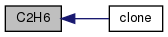## ◆ C2H6() [2/3]

 C2H6 ( const liquidProperties & l, const NSRDSfunc5 & density, const NSRDSfunc1 & vapourPressure, const NSRDSfunc6 & heatOfVapourisation, const NSRDSfunc14 & heatCapacity, const NSRDSfunc0 & enthalpy, const NSRDSfunc7 & idealGasHeatCapacity, const NSRDSfunc4 & secondVirialCoeff, const NSRDSfunc1 & dynamicViscosity, const NSRDSfunc2 & vapourDynamicViscosity, const NSRDSfunc0 & thermalConductivity, const NSRDSfunc2 & vapourThermalConductivity, const NSRDSfunc6 & surfaceTension, const APIdiffCoefFunc & vapourDiffussivity )

Construct from components.

Definition at line 87 of file C2H6.C.

## ◆ C2H6() [3/3]

 C2H6 ( const dictionary & dict )

Construct from dictionary.

Definition at line 121 of file C2H6.C.

Here is the call graph for this function: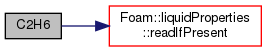## ◆ TypeName()

 TypeName ( "C2H6" )

Runtime type information.

## ◆ clone()

 virtual autoPtr clone ( ) const
inlinevirtual

Construct and return clone.

Implements liquidProperties.

Definition at line 115 of file C2H6.H.

Here is the call graph for this function: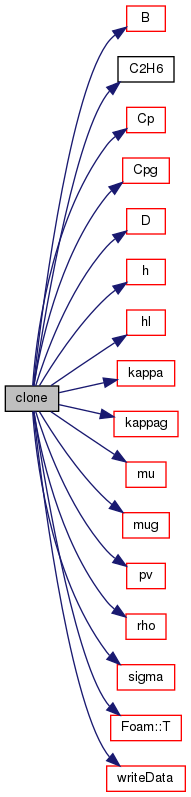## ◆ rho()

 Foam::scalar rho ( scalar p, scalar T ) const
inlinevirtual

Liquid density [kg/m^3].

Implements thermophysicalProperties.

Definition at line 26 of file C2H6I.H.

References NSRDSfunc5::f().

Referenced by C2H6::clone().

Here is the call graph for this function: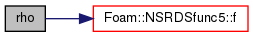Here is the caller graph for this function: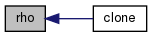## ◆ pv()

 Foam::scalar pv ( scalar p, scalar T ) const
inlinevirtual

Vapour pressure [Pa].

Implements liquidProperties.

Definition at line 32 of file C2H6I.H.

References NSRDSfunc1::f().

Referenced by C2H6::clone().

Here is the call graph for this function: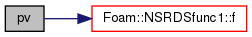Here is the caller graph for this function: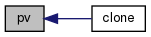## ◆ hl()

 Foam::scalar hl ( scalar p, scalar T ) const
inlinevirtual

Heat of vapourisation [J/kg].

Implements liquidProperties.

Definition at line 38 of file C2H6I.H.

References NSRDSfunc6::f().

Referenced by C2H6::clone().

Here is the call graph for this function: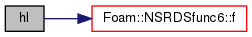Here is the caller graph for this function: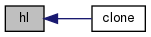## ◆ Cp()

 Foam::scalar Cp ( scalar p, scalar T ) const
inlinevirtual

Liquid heat capacity [J/(kg K)].

Implements thermophysicalProperties.

Definition at line 44 of file C2H6I.H.

References NSRDSfunc14::f().

Referenced by C2H6::clone().

Here is the call graph for this function: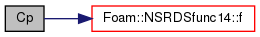Here is the caller graph for this function: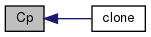## ◆ h()

 Foam::scalar h ( scalar p, scalar T ) const
inlinevirtual

Liquid Enthalpy [J/(kg)].

Implements liquidProperties.

Definition at line 50 of file C2H6I.H.

References NSRDSfunc0::f().

Referenced by C2H6::clone().

Here is the call graph for this function: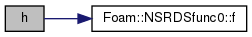Here is the caller graph for this function: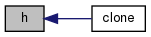## ◆ Cpg()

 Foam::scalar Cpg ( scalar p, scalar T ) const
inlinevirtual

Ideal gas heat capacity [J/(kg K)].

Implements liquidProperties.

Definition at line 56 of file C2H6I.H.

References NSRDSfunc7::f().

Referenced by C2H6::clone().

Here is the call graph for this function: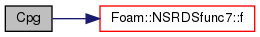Here is the caller graph for this function: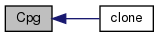## ◆ B()

 Foam::scalar B ( scalar p, scalar T ) const
inline

Second Virial Coefficient [m^3/kg].

Definition at line 62 of file C2H6I.H.

References NSRDSfunc4::f().

Referenced by C2H6::clone().

Here is the call graph for this function: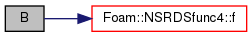Here is the caller graph for this function: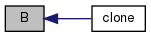## ◆ mu()

 Foam::scalar mu ( scalar p, scalar T ) const
inlinevirtual

Liquid viscosity [Pa s].

Implements liquidProperties.

Definition at line 68 of file C2H6I.H.

References NSRDSfunc1::f().

Referenced by C2H6::clone().

Here is the call graph for this function: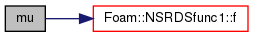Here is the caller graph for this function: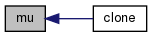## ◆ mug()

 Foam::scalar mug ( scalar p, scalar T ) const
inlinevirtual

Vapour viscosity [Pa s].

Implements liquidProperties.

Definition at line 74 of file C2H6I.H.

References NSRDSfunc2::f().

Referenced by C2H6::clone().

Here is the call graph for this function: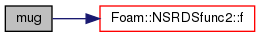Here is the caller graph for this function: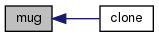## ◆ kappa()

 Foam::scalar kappa ( scalar p, scalar T ) const
inlinevirtual

Liquid thermal conductivity [W/(m K)].

Implements liquidProperties.

Definition at line 80 of file C2H6I.H.

References NSRDSfunc0::f().

Referenced by C2H6::clone().

Here is the call graph for this function: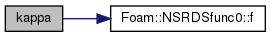Here is the caller graph for this function: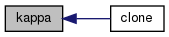## ◆ kappag()

 Foam::scalar kappag ( scalar p, scalar T ) const
inlinevirtual

Vapour thermal conductivity [W/(m K)].

Implements liquidProperties.

Definition at line 86 of file C2H6I.H.

References NSRDSfunc2::f().

Referenced by C2H6::clone().

Here is the call graph for this function: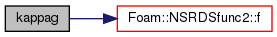Here is the caller graph for this function: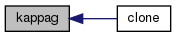## ◆ sigma()

 Foam::scalar sigma ( scalar p, scalar T ) const
inlinevirtual

Surface tension [N/m].

Implements liquidProperties.

Definition at line 92 of file C2H6I.H.

References NSRDSfunc6::f().

Referenced by C2H6::clone().

Here is the call graph for this function: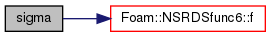Here is the caller graph for this function: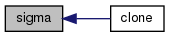## ◆ D() [1/2]

 Foam::scalar D ( scalar p, scalar T ) const
inlinevirtual

Vapour diffussivity [m2/s].

Implements liquidProperties.

Definition at line 98 of file C2H6I.H.

References APIdiffCoefFunc::f().

Referenced by C2H6::clone().

Here is the call graph for this function: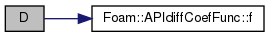Here is the caller graph for this function: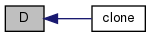## ◆ D() [2/2]

 Foam::scalar D ( scalar p, scalar T, scalar Wb ) const
inlinevirtual

Vapour diffussivity [m2/s] with specified binary pair.

Implements liquidProperties.

Definition at line 104 of file C2H6I.H.

References APIdiffCoefFunc::f().

Here is the call graph for this function:## ◆ writeData()

 void writeData ( Ostream & os ) const
virtual

Write the function coefficients.

Implements liquidProperties.

Definition at line 131 of file C2H6.C.

References liquidProperties::writeData().

Referenced by C2H6::clone(), and Foam::operator<<().

Here is the call graph for this function: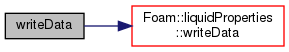Here is the caller graph for this function:## ◆ liquidProperties

 friend class liquidProperties
friend

Definition at line 81 of file C2H6.H.

## ◆ operator<<

 Ostream& operator<< ( Ostream & os, const C2H6 & l )
friend

Ostream Operator.

Referenced by C2H6::clone().

The documentation for this class was generated from the following files:
• src/thermophysicalModels/thermophysicalProperties/liquidProperties/C2H6/C2H6.H
• src/thermophysicalModels/thermophysicalProperties/liquidProperties/C2H6/C2H6.C
• src/thermophysicalModels/thermophysicalProperties/liquidProperties/C2H6/C2H6I.H